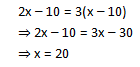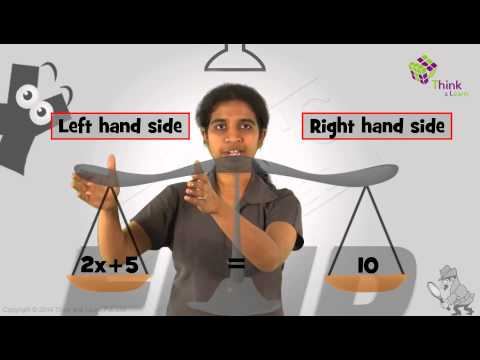# Linear Equations : Applications

There are various applications of linear equations in Mathematics as well as in real life. An algebraic equation is an equality that includes variables and equal sign (=). A linear equation is an equation of degree one.

The knowledge of mathematics is frequently applied through word problems, and the applications of linear equations are observed on a wide scale to solve such word problems. Here, we are going to discuss the linear equation applications and how to use them in the real world with the help of an example.

## What is Linear Equation?

A linear equation is an algebraic expression with a variable and equality sign (=), whose highest degree is equal to 1. For example, 2x – 1 = 5 is a linear equation.

• A linear equation with one variable and degree one is called a linear equation in one variable. (Eg, 3x + 5 = 0)
• A linear equation with degrees one and two variables is called a linear equation in two variables. (Eg, 3x + 5y = 0)

The graphical representation of linear equation is ax + by + c = 0, where,

• a and b are coefficients
• x and y are variables
• c is a constant term

## What are the Applications of Linear Equations?

In real life, the applications of linear equations are vast. To tackle real-life problems using algebra, we convert the given situation into mathematical statements in such a way that it clearly illustrates the relationship between the unknowns (variables) and the information provided. The following steps are involved while restating a situation into a mathematical statement:

• Translate the problem statement into a mathematical statement and set it up in the form of algebraic expression in a manner it illustrates the problem aptly.
• Identify the unknowns in the problem and assign variables (quantity whose value can change depending upon the mathematical context) to these unknown quantities.
• Read the problem thoroughly multiple times and cite the data, phrases and keywords. Organize the information obtained sequentially.
• Frame an equation with the help of the algebraic expression and the data provided in the problem statement and solve it using systematic techniques of equation solving.
• Retrace your solution to the problem statement and analyze if it suits the criterion of the problem.

There you go!! Using these steps and applications of linear equations word problems can be solved easily.

## Applications of Linear equations in Real life

• Finding unknown age
• Finding unknown angles in geometry
• For calculation of speed, distance or time
• Problems based on force and pressure

Let us look into an example to analyze the applications of linear equations in depth.

## Applications of Linear Equations Solved Example

Example:

Rishi is twice as old as Vani. 10 years ago his age was thrice of Vani. Find their present ages.

Solution:

In this word problem, the ages of Rishi and Vani are unknown quantities. Therefore as discussed above, let us first choose variables for the unknowns.

Let us assume that Vani’s present age is ‘x’ years. Since Rishi’s present age is 2 times that of Vani, therefore his present age can be assumed to be ‘2x’.

10 years ago, Vani’s age would have been ‘x – 10 ’, and Rishi’s age would have been ‘2x – 10’. According to the problem statement, 10 years ago, Rishi’s age was thrice of Vani, i.e. 2x – 10 = 3(x – 10).

We have our linear equation in the variable ‘x’ which clearly defines the problem statement. Now we can solve this linear equation easily and get the result.This implies that the current age of Vani is 20 years, and Rishi’s age is ‘2x,’ i.e. 40 years. Let us retrace our solution. If the present age of Vani is 20 years then 10 years ago her age would have been 10 years, and Rishi’s age would have been 30 years which satisfies our problem statement. Thus, applications of linear equations enable us to tackle such real-world problems.

## Video Lesson on Applications of Linear Equation## Frequently Asked Questions – FAQs

Q1

### What is the linear equation?

A linear equation is an algebraic equation in which each term is either a constant or the product of a constant and a variable.
For example, ax + b = 0, where a and b are constants and a ≠ 0.
Q2

### What are the applications of Linear equations in real life?

The applications of linear equations in real life are:
Finding unknown age
Finding unknown angles in geometry
For calculation of speed, distance or time
Problems based on force and pressure
Q3

### What is a linear equation with one variable?

A linear equation with one variable and degree one is called a linear equation in one variable. (Eg, 3x + 5 = 0)
Q4

### What is a linear equation with two variables?

A linear equation with degrees one and two variables is called a linear equation in two variables. (Eg, 3x + 5y = 0)
Q5

### What is a graphical representation of a linear equation?

The graphical representation of linear equation is ax + by + c = 0,
Where,
a and b are coefficients
x and y are variables
c is a constant term
Test your Knowledge on Applications of Linear equations